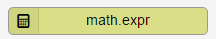# math.expr action

This trigger action will perform a definitive calculation to compute the value of a mathematical expression. Mathematical expression combines, numbers, variables, and operators to calculate the value of something.

## Action

This trigger action is found under the Math heading.## Canvas

When dragged onto the canvas, the appearance of the action is a rectangle with two routing points.The left routing point (red) is the failure route. This route will be taken if there is an error during execution.

The right routing point (green) is the success route. This route will be taken if action executes successfully.

## Form## Parameter

Name Type Required Description

Expression

String Yes This field will contain a formula to transform or manipulate data during the execution of this trigger. For example: \$(event.prop.value) / 100
Expression

Every expression consists of at least one operand and one or more operators. Operands are values, such as 0, 1, 3.14, and 1234.5678; or variables like \$(event.prop.value) and \$(action.0.value). Operators are symbols that represent particular mathematical actions.

In the expression "\$(event.prop.value) + \$(action.0.value) - 10":

• \$(event.prop.value), \$(action.0.value), and 10 are operands
• + and - are operators.

The following is a list of valid arithmetic operators:

Operator

Description

+

-

Subtraction

*

Multiplication

/

Division

^

Exponentiation (for example: 2^3 = 8)

% Modulo
@ Unary minus

( )

Force precedence of evaluation

sqrt() Square root (for example: sqrt(64) = 8)

## Action variable

Name Type Description
\$(action.X.value) Float The result of the calculations performed using the expression.

Expressions do not contain an equal sign (=) or any type of relational operation. If you are performing relational operations (>, <,==, and so on) then the output of math.expr is either 1 (True) or 0 (False).